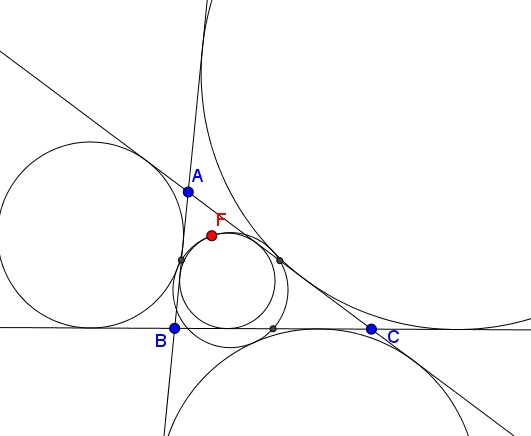## Feuerbach's Theorem: What Is It About? A Mathematical Droodle

ExplanationThis is a famous result discovered by Karl Wilhelm Feuerbach (1800-1834): the 9-point circle of a triangle touches its incircle and the three excircles. Feuerbach proved the theorem by computing algebraically the distances between the centers of the circles. Other proofs depend on the inversion in a circle. The point of tangency of the incircle and the 9-point circle is known as the Feuerbach point. The 9-point circle is known to house a few dozen other intereseting points. The points of tangency in Feuerbach's theorem are involved in two cases of incidence.### Nine Point Circle